# Price elasticity of demand (PED)

## Price elasticity of demand and its determinants

Price elasticity of demand: measures the responsiveness of quantity demanded to a change in price, along a given demand curve.

• Mathematically the value is negative, but we treat it as positive.

Price elastic demand (less than infinity).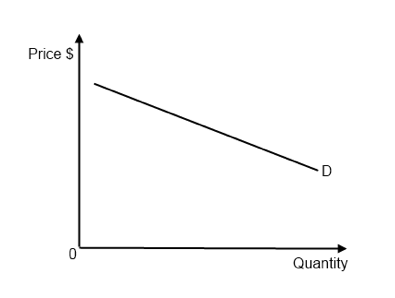Figure 2.1 - Price elastic demand

Price inelastic demand (greater than zero)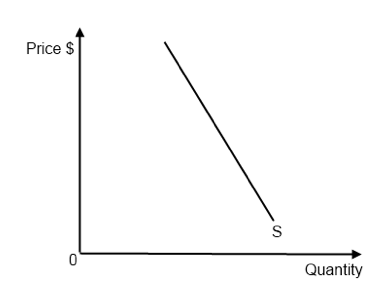Figure 2.2 - Price inelastic demand

Unit elastic demand

• %change in P = %change in Qd
• Total revenue will not change when price changes (same revenue box)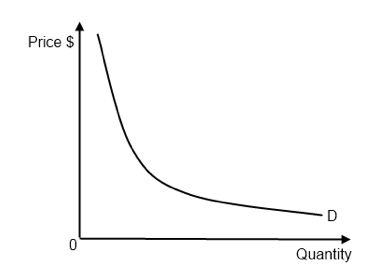Figure 2.3 - Unit elastic demand

Perfectly elastic demand, demand is zero at all but one price.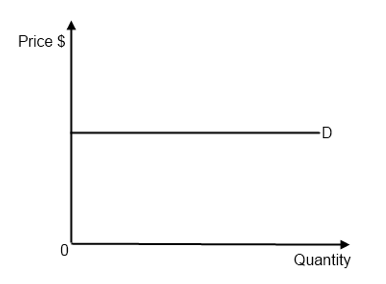Figure 2.4 - Perfectly elastic demand

Perfectly inelastic demand, demand is constant at any price.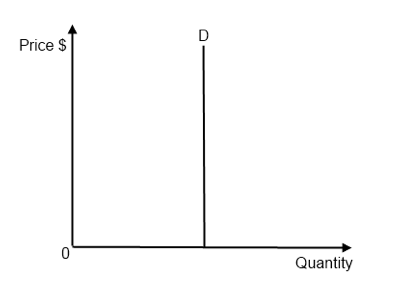Figure 2.5 - Perfectly inelastic demand

Determinants of PED:

1. Number and closeness of substitutes: more substitutes available & closer → higher PED
2. Degree of necessity (and how widely it is defined): lower the degree of necessity → higher PED; the more vague it is defined, i.e. food → higher PED
• As it is more narrowly defined → more subjective
3. Time period considered: more time to consider → higher PED
• Inelastic in the short term, elastic in the long term
4. Income spent: the higher the income spent → higher PED
• Value of PED falls as the measuring points move down a demand curve. PED is not represented by the slope of the demand curve.

## Applications of price elasticity of demand

Applications of PED:

1. Governments: if inelastic (low) PED → less consequence if P → impose more indirect tax
2. Firms: if inelastic (low) PED → more revenue if P → price of the product rises